Next: Temporal Gain Correction Up: PSPC Gain Previous: ADC Non-linearities

### Gain Saturation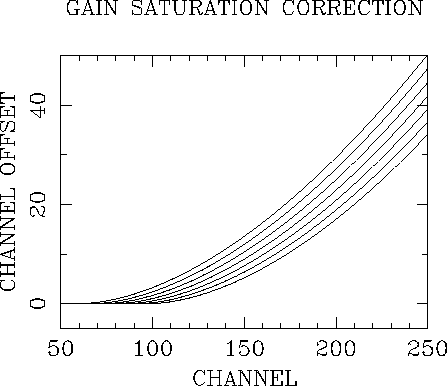Figure 3.13: The difference between the true and measured pulse height channel due to gain saturation. The curves are for nominal gains of 90 (top), 100, 110, 120, 130, 140, and 150 (bottom) [Hasinger and Snowden1990].

At large charge input, the PSPC shows some saturation of the gain (i.e., a fall-off in the signal amplification). This is a reasonably minor effect (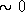% below 1keV,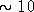% at 2keV) and has been modelled using the spectral calibration data. The gain saturation correction was determined using the same data used to create the detector response matrix.  An analytic relationship was determined between the mean of the fits to the calibration data and the true gain. (Since the Al K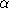calibration line is at 1487eV, it too is affected by the gain saturation.) In the SASS processing, first the channel at which gain saturation becomes significant is estimated based on the mean channel of the Al Kline.  Then, the gain correction is calculated based on the empirical relationship between gain and calibration line channel. This gain correction is later used to make the temporal correction for the entire PSPC channel range. For details of the algorithm and SASS calibration files used see Chap. 8. The nominal gain is assumed to bring the Al Kline into channel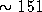. The gain saturation correction is shown in Fig. 3.13 for a range in nominal gains.

If you have problems/suggestions please send mail to rosat_svc@mpe-garching.mpg.de